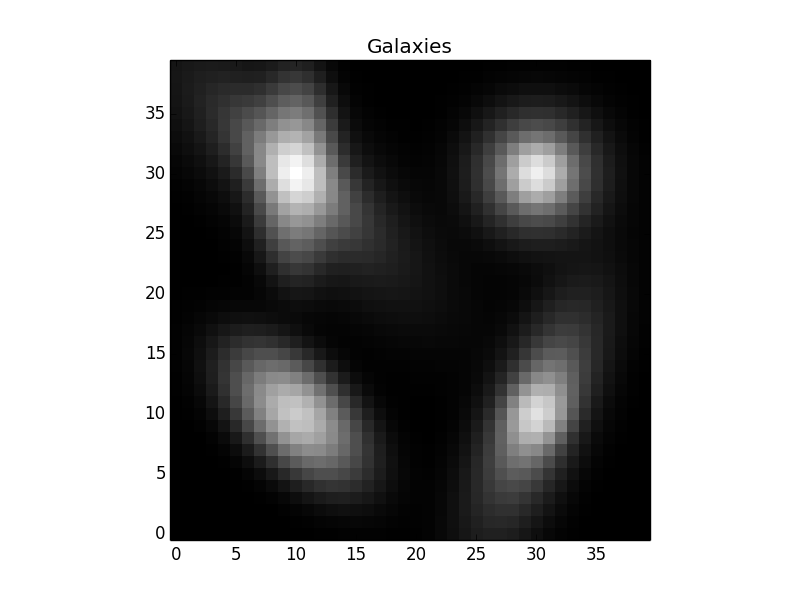# Introduction to using The Tractor (Part 2)¶

## Galaxies¶

The Tractor currently supports parametric Exponential (“exp”, ExpGalaxy) and deVaucoulers (“dev”, DevGalaxy) galaxies, composite galaxies (deV+exp, CompositeGalaxy and FixedCompositeGalaxy), and general Sersic galaxies (SersicGalaxy). The dev and exp galaxies are in the tractor.galaxy package, while Sersic is in tractor.sersic.

Each of these galaxies is described by its position, brightness, and an ellipse describing its shape. Composite galaxies have a brightness and shape for the dev and exp components. FixedComposite has a single brightness and a FracDev parameter describing how the flux is split between the exp and dev components. Sersic galaxies also a Sersic index parameter.

A number of ellipse parameterizations are available, including GalaxyShape (radius, axis ratio, and position angle; in galaxy.py), EllipseE (radius, e1, e2)—which I believe are called g1,g2 in GalSim, and EllipseESoft (log-radius, ee1, ee2), where ee1,ee2 go through a sigmoid softening function (1-exp(-|ee|)) to get to the standard e1,e2. This parameterization has the advantage that the parameter space is smooth and unbounded, which tends to make optimizers happy.

Let’s see an example of creating some Galaxy objects.

```import numpy as np
import pylab as plt
from tractor import *
from tractor.galaxy import *
from tractor.sersic import *

# size of image
W,H = 40,40

# PSF size
psfsigma = 1.

# per-pixel noise
noisesigma = 0.01

# create tractor.Image object for rendering synthetic galaxy
# images
tim = Image(data=np.zeros((H,W)), invvar=np.ones((H,W)) / (noisesigma**2),
psf=NCircularGaussianPSF([psfsigma], [1.]))

sources = [ ExpGalaxy(PixPos(10,10), Flux(10.), GalaxyShape(3., 0.5, 45.)),
CompositeGalaxy(PixPos(10,30),
Flux(10.), EllipseE(3., 0.5, 0.),
Flux(10.), EllipseE(3., 0., -0.5)),
SersicGalaxy(PixPos(30,10), Flux(10.),
EllipseESoft(1., 0.5, 0.5), SersicIndex(3.)),
FixedCompositeGalaxy(PixPos(30,30), Flux(10.), 0.8,
EllipseE(2., 0., 0.),EllipseE(1., 0., 0.))]

tractor = Tractor([tim], sources)

mod = tractor.getModelImage(0)

# Plot
plt.clf()
plt.imshow(np.log(mod + noisesigma),
interpolation='nearest', origin='lower', cmap='gray')
plt.title('Galaxies')
plt.savefig('7.png')
```

Notice that we can mix-and-match different ellipse types. The image looks like: